SLVSBN0C March   2013  – March 2017

PRODUCTION DATA.

1. Features
2. Applications
3. Description
4. Revision History
5. Pin Configuration and Functions
6. Specifications
7. Detailed Description
8. Application and Implementation
1. 8.1 Application Information
2. 8.2 Typical Application
1. 8.2.1 Design Requirements
2. 8.2.2 Detailed Design Procedure
3. 8.2.3 Application Curves
3. 8.3 Inverting Power
4. 8.4 Split Rail Power Supply
9. Power Supply Recommendations
10. 10Layout
1. 10.1 Layout Guidelines
2. 10.2 Layout Examples
11. 11Device and Documentation Support
12. 12Mechanical, Packaging, and Orderable Information

• DDA|8
• DDA|8

## 8 Application and Implementation

NOTE

Information in the following applications sections is not part of the TI component specification, and TI does not warrant its accuracy or completeness. TI’s customers are responsible for determining suitability of components for their purposes. Customers should validate and test their design implementation to confirm system functionality.

### 8.1 Application Information

The TPS54560 is a 60 V, 5 A, step down regulator with an integrated high side MOSFET. Idea applications are: 12 V, 24 V and 48 V Industrial, automotive and communications power systems

### 8.2 Typical Application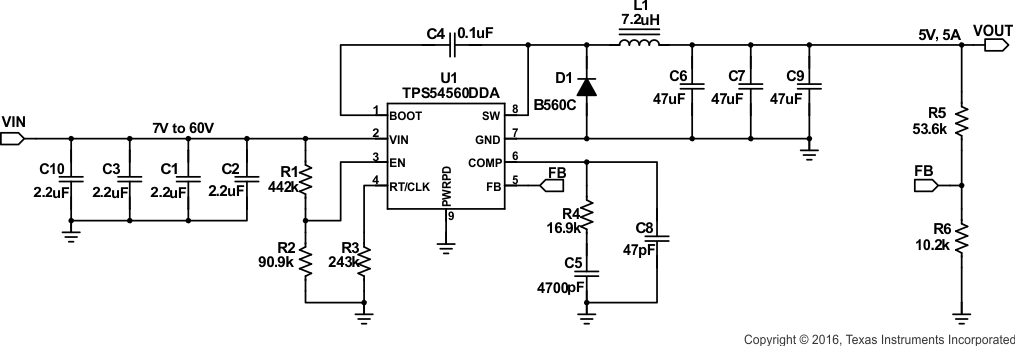Figure 33. 5 V Output TPS54560 Design Example

#### 8.2.1 Design Requirements

This guide illustrates the design of a high frequency switching regulator using ceramic output capacitors. A few parameters must be known in order to start the design process. These requirements are typically determined at the system level. Calculations can be done with the aid of WEBENCH or the excel spreadsheet (SLVC452) located on the product page. For this example, start with the following known parameters:

### Table 1. Design Parameters

DESIGN PARAMETERS EXAMPLE VALUES
Output Voltage 5 V
Transient Response 1.25 A to 3.75 A load step ΔVOUT = 4 %
Maximum Output Current 5 A
Input Voltage 12 V nom. 7 V to 60 V
Output Voltage Ripple 0.5% of VOUT
Start Input Voltage (rising VIN) 6.5 V
Stop Input Voltage (falling VIN) 5 V

#### 8.2.2.1 Custom Design with WEBENCH® Tools

Click here to create a custom design using the TPS54560 device with the WEBENCH® Power Designer.

1. Start by entering your VIN, VOUT, and IOUT requirements.
2. Optimize your design for key parameters like efficiency, footprint and cost using the optimizer dial and compare this design with other possible solutions from Texas Instruments.
3. The WEBENCH Power Designer provides you with a customized schematic along with a list of materials with real time pricing and component availability.
4. In most cases, you will also be able to:
• Run electrical simulations to see important waveforms and circuit performance
• Run thermal simulations to understand the thermal performance of your board
• Print PDF reports for the design, and share your design with colleagues

#### 8.2.2.2 Selecting the Switching Frequency

The first step is to choose a switching frequency for the regulator. Typically, the designer uses the highest switching frequency possible since this produces the smallest solution size. High switching frequency allows for lower value inductors and smaller output capacitors compared to a power supply that switches at a lower frequency. The switching frequency that can be selected is limited by the minimum on-time of the internal power switch, the input voltage, the output voltage and the frequency foldback protection.

Equation 9 and Equation 10 should be used to calculate the upper limit of the switching frequency for the regulator. Choose the lower value result from the two equations. Switching frequencies higher than these values results in pulse skipping or the lack of overcurrent protection during a short circuit.

The typical minimum on time, tonmin, is 135 ns for the TPS54560. For this example, the output voltage is 5 V and the maximum input voltage is 60 V, which allows for a maximum switch frequency up to 708 kHz to avoid pulse skipping from Equation 9. To ensure overcurrent runaway is not a concern during short circuits use Equation 10 to determine the maximum switching frequency for frequency foldback protection. With a maximum input voltage of 60 V, assuming a diode voltage of 0.7 V, inductor resistance of 11 mΩ, switch resistance of 92 mΩ, a current limit value of 6 A and short circuit output voltage of 0.1 V, the maximum switching frequency is 855 kHz.

For this design, a lower switching frequency of 400 kHz is chosen to operate comfortably below the calculated maximums. To determine the timing resistance for a given switching frequency, use Equation 7 or the curve in Figure 6. The switching frequency is set by resistor R3 shown in Figure 33. For 400 kHz operation, the closest standard value resistor is 243 kΩ.

Equation 25.Equation 26.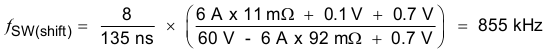Equation 27.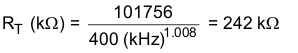#### 8.2.2.3 Output Inductor Selection (LO)

To calculate the minimum value of the output inductor, use Equation 28.

KIND is a ratio that represents the amount of inductor ripple current relative to the maximum output current. The inductor ripple current is filtered by the output capacitor. Therefore, choosing high inductor ripple currents impacts the selection of the output capacitor since the output capacitor must have a ripple current rating equal to or greater than the inductor ripple current. In general, the inductor ripple value is at the discretion of the designer, however, the following guidelines may be used.

For designs using low ESR output capacitors such as ceramics, a value as high as KIND = 0.3 may be desirable. When using higher ESR output capacitors, KIND = 0.2 yields better results. Since the inductor ripple current is part of the current mode PWM control system, the inductor ripple current should always be greater than 150 mA for stable PWM operation. In a wide input voltage regulator, it is best to choose relatively large inductor ripple current. This provides sufficienct ripple current with the input voltage at the minimum.

For this design example, KIND = 0.3 and the inductor value is calculated to be 7.6 μH. The nearest standard value is 7.2 μH. It is important that the RMS current and saturation current ratings of the inductor not be exceeded. The RMS and peak inductor current can be found from Equation 30 and Equation 31. For this design, the RMS inductor current is 5 A and the peak inductor current is 5.8 A. The chosen inductor is a WE 7447798720, which has a saturation current rating of 7.9 A and an RMS current rating of 6 A.

As the equation set demonstrates, lower ripple currents will reduce the output voltage ripple of the regulator but will require a larger value of inductance. Selecting higher ripple currents will increase the output voltage ripple of the regulator but allow for a lower inductance value.

The current flowing through the inductor is the inductor ripple current plus the output current. During power up, faults or transient load conditions, the inductor current can increase above the peak inductor current level calculated above. In transient conditions, the inductor current can increase up to the switch current limit of the device. For this reason, the most conservative design approach is to choose an inductor with a saturation current rating equal to or greater than the switch current limit of the TPS54560 which is nominally 7.5 A.

Equation 28.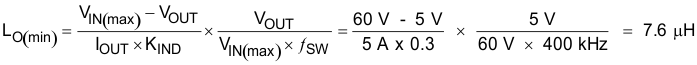spacer

Equation 29.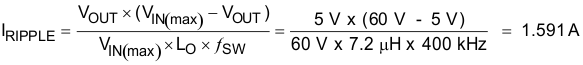spacer

Equation 30.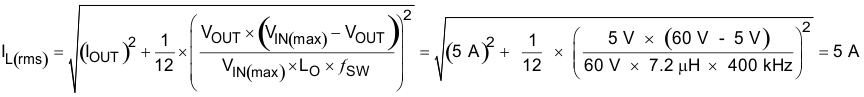spacer

Equation 31.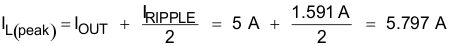#### 8.2.2.4 Output Capacitor

There are three primary considerations for selecting the value of the output capacitor. The output capacitor determines the modulator pole, the output voltage ripple, and how the regulator responds to a large change in load current. The output capacitance needs to be selected based on the most stringent of these three criteria.

The output capacitor must also be sized to absorb energy stored in the inductor when transitioning from a high to low load current. The catch diode of the regulator can not sink current so energy stored in the inductor can produce an output voltage overshoot when the load current rapidly decreases. A typical load step response is shown in Figure 34. The excess energy absorbed in the output capacitor will increase the voltage on the capacitor. The capacitor must be sized to maintain the desired output voltage during these transient periods. Equation 33 calculates the minimum capacitance required to keep the output voltage overshoot to a desired value, where LO is the value of the inductor, IOH is the output current under heavy load, IOL is the output under light load, Vf is the peak output voltage, and Vi is the initial voltage. For this example, the worst case load step will be from 3.75 A to 1.25 A. The output voltage increases during this load transition and the stated maximum in our specification is 4 % of the output voltage. This makes Vf = 1.04 × 5 = 5.2. Vi is the initial capacitor voltage which is the nominal output voltage of 5 V. Using these numbers in Equation 33 yields a minimum capacitance of
44.1 μF.

Equation 34 calculates the minimum output capacitance needed to meet the output voltage ripple specification, where ƒsw is the switching frequency, VORIPPLE is the maximum allowable output voltage ripple, and IRIPPLE is the inductor ripple current. Equation 34 yields 19.9 μF.

Equation 35 calculates the maximum ESR an output capacitor can have to meet the output voltage ripple specification. Equation 35 indicates the ESR should be less than 15.7 mΩ.

The most stringent criteria for the output capacitor is 62.5 μF required to maintain the output voltage within regulation tolerance during a load transient.

Capacitance de-ratings for aging, temperature and dc bias increases this minimum value. For this example, 3 x 47 μF, 10 V ceramic capacitors with 5 mΩ of ESR will be used. The derated capacitance is 87.4 µF, well above the minimum required capacitance of 62.5 µF.

Capacitors are generally rated for a maximum ripple current that can be filtered without degrading capacitor reliability. Some capacitor data sheets specify the Root Mean Square (RMS) value of the maximum ripple current. Equation 36 can be used to calculate the RMS ripple current that the output capacitor must support. For this example, Equation 36 yields 459 mA.

Equation 32.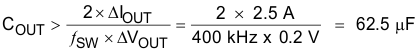Equation 33.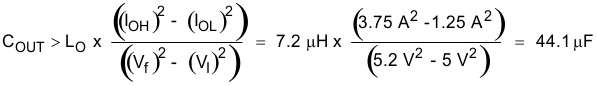Equation 34.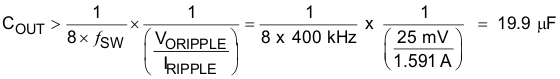Equation 35.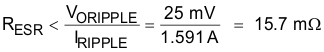Equation 36.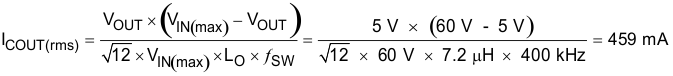#### 8.2.2.5 Catch Diode

The TPS54560 requires an external catch diode between the SW terminal and GND. The selected diode must have a reverse voltage rating equal to or greater than VIN(max). The peak current rating of the diode must be greater than the maximum inductor current. Schottky diodes are typically a good choice for the catch diode due to their low forward voltage. The lower the forward voltage of the diode, the higher the efficiency of the regulator.

Typically, diodes with higher voltage and current ratings have higher forward voltages. A diode with a minimum of 60 V reverse voltage is preferred to allow input voltage transients up to the rated voltage of the TPS54560.

For the example design, the B560C-13-F Schottky diode is selected for its lower forward voltage and good thermal characteristics compared to smaller devices. The typical forward voltage of the B560C-13-F is 0.70 volts at 5 A.

The diode must also be selected with an appropriate power rating. The diode conducts the output current during the off-time of the internal power switch. The off-time of the internal switch is a function of the maximum input voltage, the output voltage, and the switching frequency. The output current during the off-time is multiplied by the forward voltage of the diode to calculate the instantaneous conduction losses of the diode. At higher switching frequencies, the ac losses of the diode need to be taken into account. The ac losses of the diode are due to the charging and discharging of the junction capacitance and reverse recovery charge. Equation 37 is used to calculate the total power dissipation, including conduction losses and ac losses of the diode.

The B560C-13-F diode has a junction capacitance of 300 pF. Using Equation 37, the total loss in the diode at the maximum input voltage is 3.43 Watts.

If the power supply spends a significant amount of time at light load currents or in sleep mode, consider using a diode which has a low leakage current and slightly higher forward voltage drop.

Equation 37.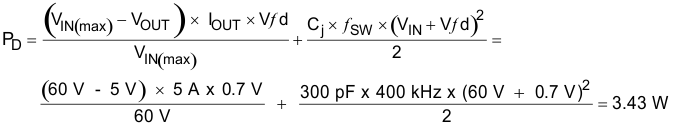#### 8.2.2.6 Input Capacitor

The TPS54560 requires a high quality ceramic type X5R or X7R input decoupling capacitor with at least 3 μF of effective capacitance. Some applications will benefit from additional bulk capacitance. The effective capacitance includes any loss of capacitance due to dc bias effects. The voltage rating of the input capacitor must be greater than the maximum input voltage. The capacitor must also have a ripple current rating greater than the maximum input current ripple of the TPS54560. The input ripple current can be calculated using Equation 38.

The value of a ceramic capacitor varies significantly with temperature and the dc bias applied to the capacitor. The capacitance variations due to temperature can be minimized by selecting a dielectric material that is more stable over temperature. X5R and X7R ceramic dielectrics are usually selected for switching regulator capacitors because they have a high capacitance to volume ratio and are fairly stable over temperature. The input capacitor must also be selected with consideration for the dc bias. The effective value of a capacitor decreases as the dc bias across a capacitor increases.

For this example design, a ceramic capacitor with at least a 60 V voltage rating is required to support the maximum input voltage. Common standard ceramic capacitor voltage ratings include 4 V, 6.3 V, 10 V, 16 V, 25 V, 50 V or 100 V. For this example, four 2.2 μF, 100 V capacitors in parallel are used. Table 2 shows several choices of high voltage capacitors.

The input capacitance value determines the input ripple voltage of the regulator. The input voltage ripple can be calculated using Equation 39. Using the design example values, IOUT = 5 A, CIN = 8.8 μF, ƒsw = 400 kHz, yields an input voltage ripple of 355 mV and a rms input ripple current of 2.26 A.

Equation 38.Equation 39.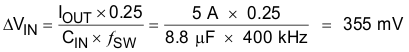### Table 2. Capacitor Types

VALUE (μF) EIA Size VOLTAGE DIALECTRIC COMMENTS
1 to 2.2 1210 100 V X7R GRM32 series
1 to 4.7 50 V
1 1206 100 V GRM31 series
1 to 2.2 50 V
1 to 1.8 2220 50 V VJ X7R series
1 to 1.2 100 V
1 to 3.9 2225 50 V
1 to 1.8 100 V
1 to 2.2 1812 100 V C series C4532
1.5 to 6.8 50 V
1 to 2.2 1210 100 V C series C3225
1 to 3.3 50 V
1 to 4.7 1210 50 V X7R dielectric series
1 100 V
1 to 4.7 1812 50 V
1 to 2.2 100 V

#### 8.2.2.7 Bootstrap Capacitor Selection

A 0.1-μF ceramic capacitor must be connected between the BOOT and SW terminals for proper operation. A ceramic capacitor with X5R or better grade dielectric is recommended. The capacitor should have a 10 V or higher voltage rating.

#### 8.2.2.8 Undervoltage Lockout Set Point

The Undervoltage Lockout (UVLO) can be adjusted using an external voltage divider on the EN terminal of the TPS54560. The UVLO has two thresholds, one for power up when the input voltage is rising and one for power down or brown outs when the input voltage is falling. For the example design, the supply should turn on and start switching once the input voltage increases above 6.5 V (UVLO start). After the regulator starts switching, it should continue to do so until the input voltage falls below 5 V (UVLO stop).

Programmable UVLO threshold voltages are set using the resistor divider of RUVLO1 and RUVLO2 between Vin and ground connected to the EN terminal. Equation 4 and Equation 5 calculate the resistance values necessary. For the example application, a 442 kΩ between VIN and EN (RUVLO1) and a 90.9 kΩ between EN and ground (RUVLO2) are required to produce the 6.5 V and 5 V start and stop voltages.

Equation 40.Equation 41.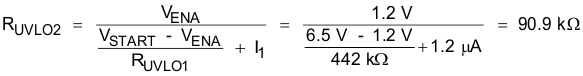#### 8.2.2.9 Output Voltage and Feedback Resistors Selection

The voltage divider of R5 and R6 sets the output voltage. For the example design, 10.2 kΩ was selected for R6. Using Equation 3, R5 is calculated as 53.5 kΩ. The nearest standard 1% resistor is 53.6 kΩ. Due to the input current of the FB terminal, the current flowing through the feedback network should be greater than 1 μA to maintain the output voltage accuracy. This requirement is satisfied if the value of R6 is less than 800 kΩ. Choosing higher resistor values decreases quiescent current and improves efficiency at low output currents but may also introduce noise immunity problems.

Equation 42.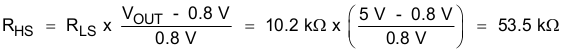#### 8.2.2.10 Minimum Input Voltage, VIN

To ensure proper operation of the device and to keep the output voltage in regulation, the input voltage at the device must be above the value calculated with Equation 43. Using the typical values for the RDS(on), Rdc and VF in this application example, the minimum input voltage is 5.71 V. The BOOT-SW = 3 V curve in Figure 1 was used for RHS = 0.12 Ω because the device will be operating with low drop out. When operating with low dropout, the BOOT-SW voltage is regulated at a lower voltage because the BOOT-SW capacitor is not refreshed every switching cycle. In the final application, the values of RDS(on), Rdc and VF used in this equation must include tolerance of the component specifications and the variation of these specifications at their maximum operating temperature in the application.

Equation 43.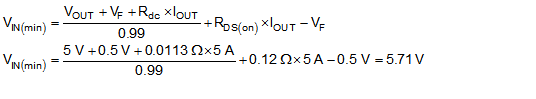#### 8.2.2.11 Compensation

There are several methods to design compensation for DC-DC regulators. The method presented here is easy to calculate and ignores the effects of the slope compensation that is internal to the device. Since the slope compensation is ignored, the actual crossover frequency will be lower than the crossover frequency used in the calculations. This method assumes the crossover frequency is between the modulator pole and the ESR zero and the ESR zero is at least 10 times greater the modulator pole.

To get started, the modulator pole, ƒp(mod), and the ESR zero, ƒz1 must be calculated using Equation 44 and Equation 45. For COUT, use a derated value of 87.4 μF. Use equations Equation 46 and Equation 47 to estimate a starting point for the crossover frequency, ƒco. For the example design, ƒp(mod) is 1821 Hz and ƒz(mod) is 1100 kHz. Equation 45 is the geometric mean of the modulator pole and the ESR zero and Equation 47 is the mean of modulator pole and half of the switching frequency. Equation 46 yields 44.6 kHz and Equation 47 gives 19.1 kHz. Use the geometric mean value of Equation 46 and Equation 47 for an initial crossover frequency. For this example, after lab measurement, the crossover frequency target was increased to 30 kHz for an improved transient response.

Next, the compensation components are calculated. A resistor in series with a capacitor is used to create a compensating zero. A capacitor in parallel to these two components forms the compensating pole.

Equation 44.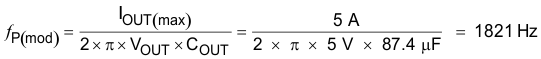Equation 45.Equation 46.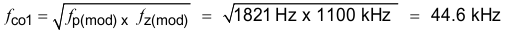Equation 47.To determine the compensation resistor, R4, use Equation 48. Assume the power stage transconductance, gmps, is 17 A/V. The output voltage, VO, reference voltage, VREF, and amplifier transconductance, gmea, are 5 V, 0.8 V and 350 μA/V, respectively. R4 is calculated to be 16.8 kΩ and a standard value of 16.9 kΩ is selected. Use Equation 49 to set the compensation zero to the modulator pole frequency. Equation 49 yields 5172 pF for compensating capacitor C5. 4700 pF is used for this design.

Equation 48.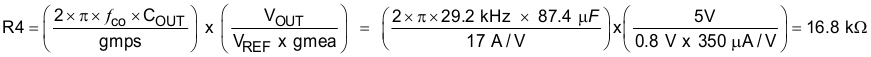Equation 49.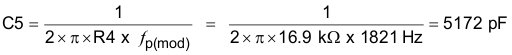A compensation pole can be implemented if desired by adding capacitor C8 in parallel with the series combination of R4 and C5. Use the larger value calculated from Equation 50 and Equation 51 for C8 to set the compensation pole. The selected value of C8 is 47 pF for this design example.

Equation 50.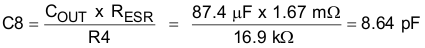Equation 51.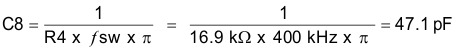#### 8.2.2.12 Discontinuous Conduction Mode and Eco-mode Boundary

With an input voltage of 12 V, the power supply enters discontinuous conduction mode when the output current is less than 408 mA. The power supply enters Eco-mode when the output current is lower than 25.3 mA. The input current draw is 257 μA with no load.

#### 8.2.2.13 Power Dissipation Estimate

The following formulas show how to estimate the TPS54560 power dissipation under continuous conduction mode (CCM) operation. These equations should not be used if the device is operating in discontinuous conduction mode (DCM).

The power dissipation of the IC includes conduction loss (PCOND), switching loss (PSW), gate drive loss (PGD) and supply current (PQ). Example calculations are shown with the 12 V typical input voltage of the design example.

Equation 52.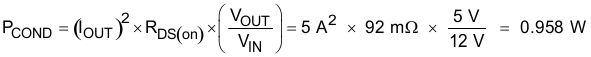spacer

Equation 53.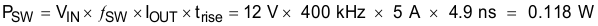spacer

Equation 54.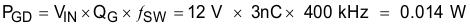spacer

Equation 55.where

• IOUT is the output current (A).
• RDS(on) is the on-resistance of the high-side MOSFET (Ω)
• VOUT is the output voltage (V).
• VIN is the input voltage (V).
• fsw is the switching frequency (Hz)
• trise is the SW terminal voltage rise time and can be estimated by trise = VIN x 0.16 ns/V + 3 ns
• QG is the total gate charge of the internal MOSFET
• IQ is the operating nonswitching supply current

Therefore,

Equation 56.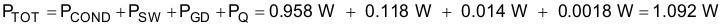For given TA,

Equation 57.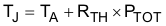For given TJMAX = 150°C

Equation 58.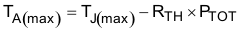where

• Ptot is the total device power dissipation (W)
• TA is the ambient temperature (°C).
• TJ is the junction temperature (°C).
• RTH is the thermal resistance of the package (°C/W)
• TJMAX is maximum junction temperature (°C)
• TAMAX is maximum ambient temperature (°C).

There will be additional power losses in the regulator circuit due to the inductor ac and dc losses, the catch diode and PCB trace resistance impacting the overall efficiency of the regulator.

#### 8.2.3 Application Curves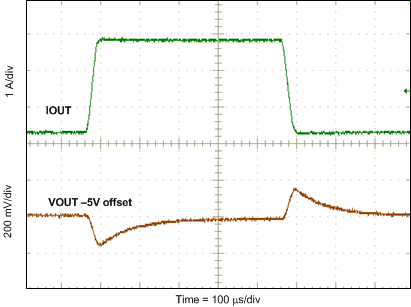Figure 34. Load Transient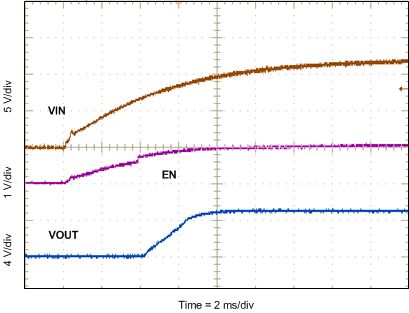Figure 36. Start-up With VIN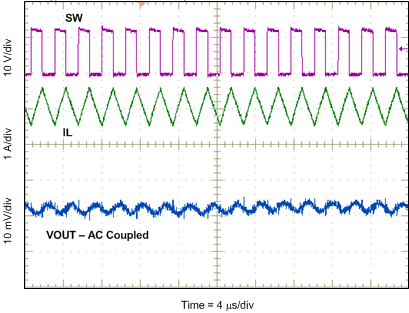Figure 38. Output Ripple CCM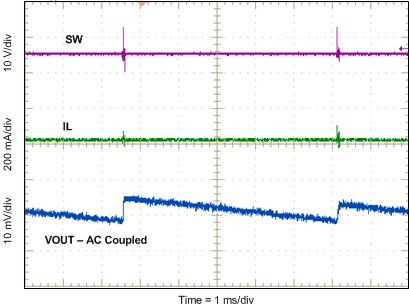Figure 40. Output Ripple PSM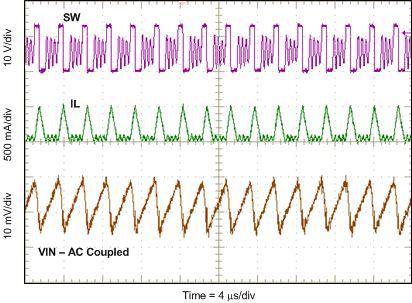IOUT = 100 mA
Figure 42. Input Ripple DCM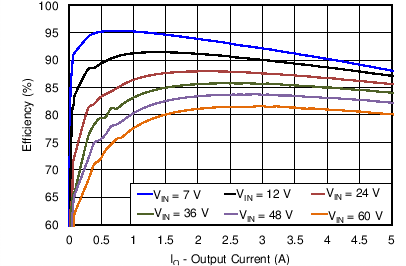VOUT = 5 V ƒsw = 400 kHz
Figure 44. Efficiency vs Load Current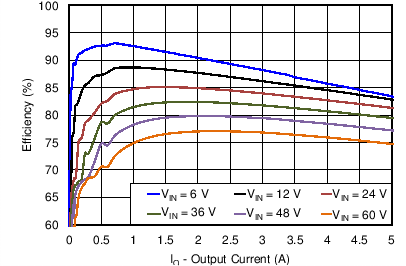VOUT = 3.3 V ƒsw = 400 kHz
Figure 46. Efficiency vs Load Current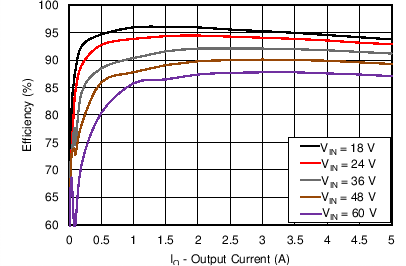V = 12 V
Figure 48. Efficiency vs Output Current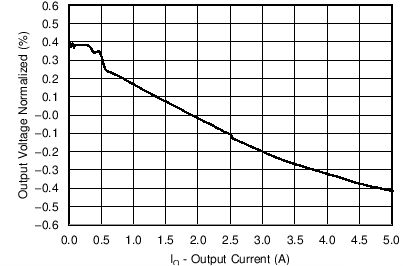VIN = 12 V VOUT = 5 V ƒsw = 400 kHz
Figure 50. Regulation vs Load Current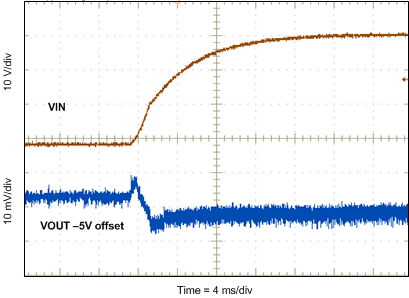Figure 35. Line Transient (8 V to 40 V)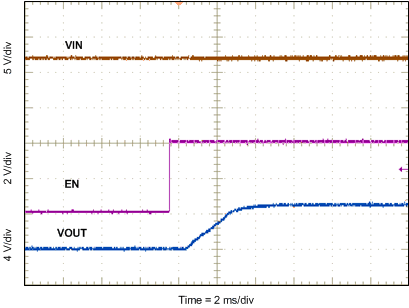Figure 37. Start-up With EN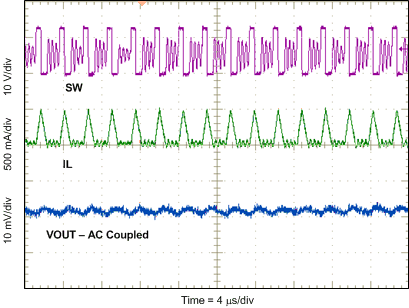IOUT = 100 mA
Figure 39. Output Ripple DCM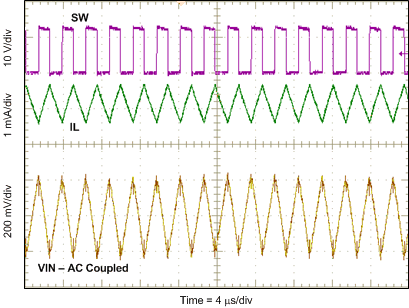Figure 41. Input Ripple CCMFigure 43. Low Dropout Operation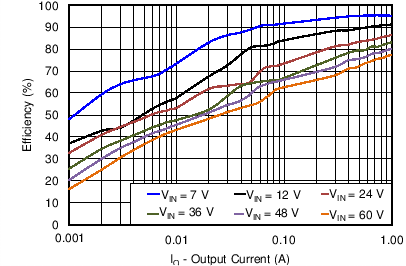VOUT = 5 V ƒsw = 400 kHz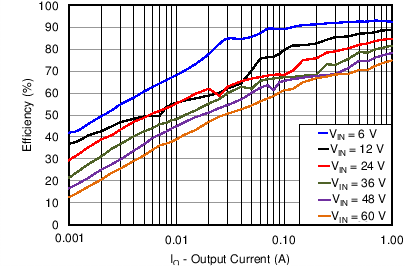VOUT = 3.3 V ƒsw = 400 kHz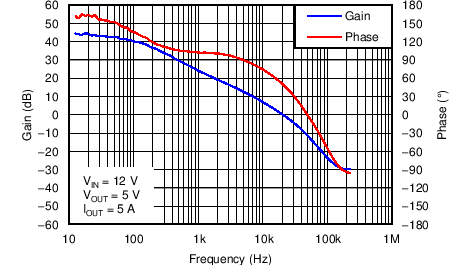VIN = 12 V VOUT = 5 V IOUT = 5 A
Figure 49. Overall Loop Frequency ResponseVOUT = 5 V IOUT = 5 A ƒsw = 400 kHz
Figure 51. Regulation vs Input Voltage

#### 8.2.3.1 Safe Operating Area

The safe operating area (SOA) of the device is shown in Figure 52, through Figure 55 for 3.3 V, 5 V and 12 V outputs and varying amounts of forced air flow. The temperature derating curves represent the conditions at which the internal and external components are at or below the manufacturer’s maximum operating temperatures. Derating limits apply to devices soldered directly to a double-sided PCB with 2 oz. copper, similar to the EVM. Careful attention must be paid to the other components chosen for the design, especially the catch diode. In most of these test conditions, the thermal performance is limited by the catch diode. When operating at high duty cycles or at higher switching frequency the TPS54560 thermal performance can become the limiting factor.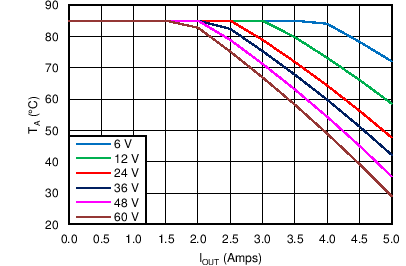Figure 52. 3.3 V Outputsƒsw = 800 kHz
Figure 54. 12 V Outputs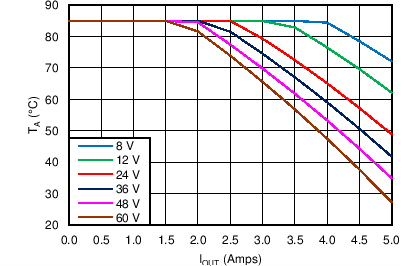Figure 53. 5 V Outputs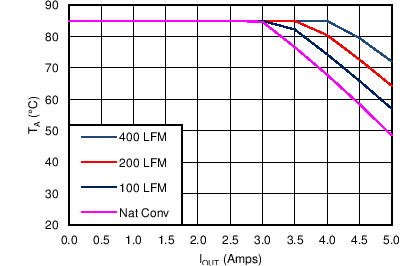ƒsw = 800 kHz
Figure 55. Air Flow Conditions
VIN = 36 V, VO = 12 V

### 8.3 Inverting Power

The TPS54560can be used to convert a positive input voltage to a negative output voltage. Idea applications are amplifiers requiring a negative power supply. For a more detailed example see SLVA317.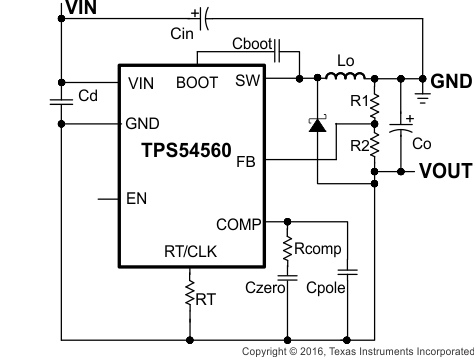Figure 56. TPS54560 Inverting Power Supply from SLVA317 Application Note

### 8.4 Split Rail Power Supply

The TPS54560 can be used to convert a positive input voltage to a split rail positive and negative output voltage by using a coupled inductor. Idea applications are amplifiers requiring a split rail positive and negative voltage power supply. For a more detailed example see SLVA369.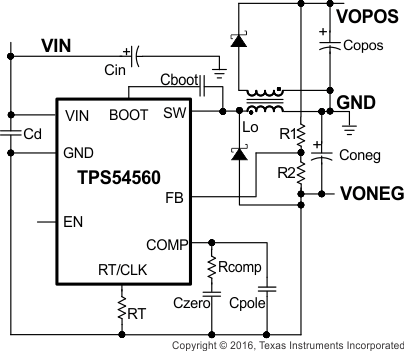Figure 57. TPS54560 Split Rail Power Supply